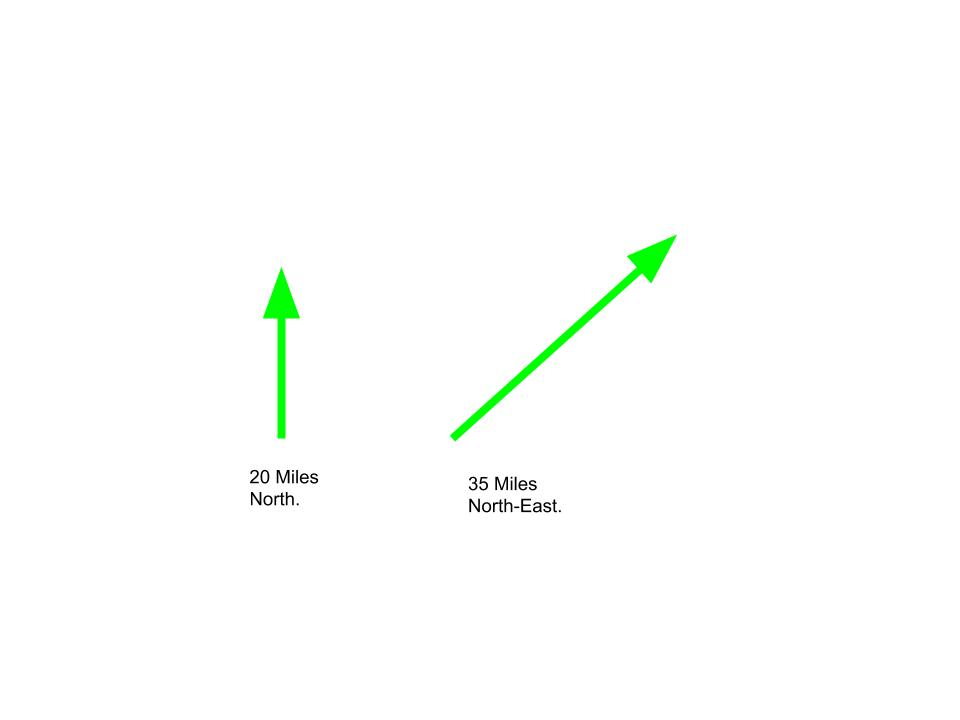GFG App
Open AppBrowser
Continue

# Using class to implement Vector Quantities in C++

A Vector Quantity is a Quantity which possesses both magnitude as well as direction. Here, magnitude is simply the amount or size of the quantity and direction is where the quantity is headed. For example, consider the statement “Go 20 miles north”. In the above statement 20 is the magnitude and North is the direction.Examples:

```Input : Store and display vector with components 3, 4, 5.
Output : 3i + 4j + 5k

Input : Dot Product for V1 = (1, 3, 5), V2 = (2, 3, 0)
Output : 11

where i, j, k are unit vectors in x, y and z directions respectively.```

In General a Vector is represented as:

V = Xi + Yj + Zk Where, X, Y and Z are the magnitude of the vector V in the directions i , j and k respectively.

Various operations that can be performed on Vectors:

1. Addition of vectors: Addition of vectors is done by adding the corresponding X, Y and Z magnitudes of the two vectors to get the resultant vector. Example: v1 = 1i + 2j + 3k v2 = 3i + 2j + 1k Therefore the resultant vector, v = v1 + v2 = 4i + 4j + 4k
2. Dot product of vectors: Dot product of two vectors v1 and v2 is calculated as:
```v = v1 . v2
= magnitude(v1)*magnitude(v2)*Cos(θ)

Where, θ is the angle between the vectors v1 and v2.```
1. Example: v1 = 1i + 2j + 3k v2 = 3i + 2j + 1k Therefore, v = v1 . v2 = 3 + 4 + 3 = 10
2. Cross product of vectors: Cross product of vectors is done by using determinant for vectors a = axi + ayj + azk and b = bxi + byj + bzk c = a X b = i(ay*bz – by*az) – j(ax*bz – az*bx) + k(ax*by – bx*ay) Example: v1 = 3i + 4j + 2k v2 = 6i + 3j + 9k Therefore, v = v1 X v2 = 30i – 15j – 15k

Below is the implementation of above operations using class in C++:

## CPP

 `#include ` `#include ` `using` `namespace` `std;`   `class` `Vector {` `private``:` `    ``int` `x, y, z;` `    ``// Components of the Vector`   `public``:` `    ``Vector(``int` `x, ``int` `y, ``int` `z)` `    ``{` `        ``// Constructor` `        ``this``->x = x;` `        ``this``->y = y;` `        ``this``->z = z;` `    ``}` `    ``Vector operator+(Vector v); ``// ADD 2 Vectors` `    ``Vector operator-(Vector v); ``// Subtraction` `    ``int` `operator^(Vector v); ``// Dot Product` `    ``Vector operator*(Vector v); ``// Cross Product` `    ``float` `magnitude()` `    ``{` `        ``return` `sqrt``(``pow``(x, 2) + ``pow``(y, 2) + ``pow``(z, 2));` `    ``}` `    ``friend` `ostream& operator<<(ostream& out, ``const` `Vector& v);` `    ``// To output the Vector` `};`   `// Addition of vectors` `Vector Vector::operator+(Vector v)` `{` `    ``int` `x1, y1, z1;` `    ``x1 = x + v.x;` `    ``y1 = y + v.y;` `    ``z1 = z + v.z;` `    ``return` `Vector(x1, y1, z1);` `}`   `// Subtraction of vectors` `Vector Vector::operator-(Vector v)` `{` `    ``int` `x1, y1, z1;` `    ``x1 = x - v.x;` `    ``y1 = y - v.y;` `    ``z1 = z - v.z;` `    ``return` `Vector(x1, y1, z1);` `}`   `// Dot product of vectors` `int` `Vector::operator^(Vector v)` `{` `    ``int` `x1, y1, z1;` `    ``x1 = x * v.x;` `    ``y1 = y * v.y;` `    ``z1 = z * v.z;` `    ``return` `(x1 + y1 + z1);` `}`   `// Cross product of vectors` `Vector Vector::operator*(Vector v)` `{` `    ``int` `x1, y1, z1;` `    ``x1 = y * v.z - z * v.y;` `    ``y1 = z * v.x - x * v.z;` `    ``z1 = x * v.y - y * v.x;` `    ``return` `Vector(x1, y1, z1);` `}`   `// Display Vector` `ostream& operator<<(ostream& out, ``const` `Vector& v)` `{` `    ``out << v.x << "i ";` `    ``if` `(v.y >= 0)` `        ``out << "+ ";` `    ``out << v.y << "j ";` `    ``if` `(v.z >= 0)` `        ``out << "+ ";` `    ``out << v.z << "k" << endl;` `    ``return` `out;` `}`   `// Driver program` `int` `main()` `{` `    ``// Let us Take the vector quantities :` `    ``// V1 = 3i + 4j + 2k` `    ``// V2 = 6i + 3j + 9k` `    ``Vector V1(3, 4, 2), V2(6, 3, 9);`   `    ``cout << "V1 = " << V1;` `    ``cout << "V2 = " << V2;`   `    ``cout << "V1 + V2 = " << (V1 + V2);` `    ``cout << "Dot Product is : " << (V1 ^ V2);` `    ``cout << "Cross Product is : " << (V1 * V2);`   `    ``return` `0;` `}`

Output:

```V1 = 3i + 4j + 2k
V2 = 6i + 3j + 9k
V1 + V2 = 9i + 7j + 11k
Dot Product is : 48Cross Product is : 30i -15j -15k```

My Personal Notes arrow_drop_up